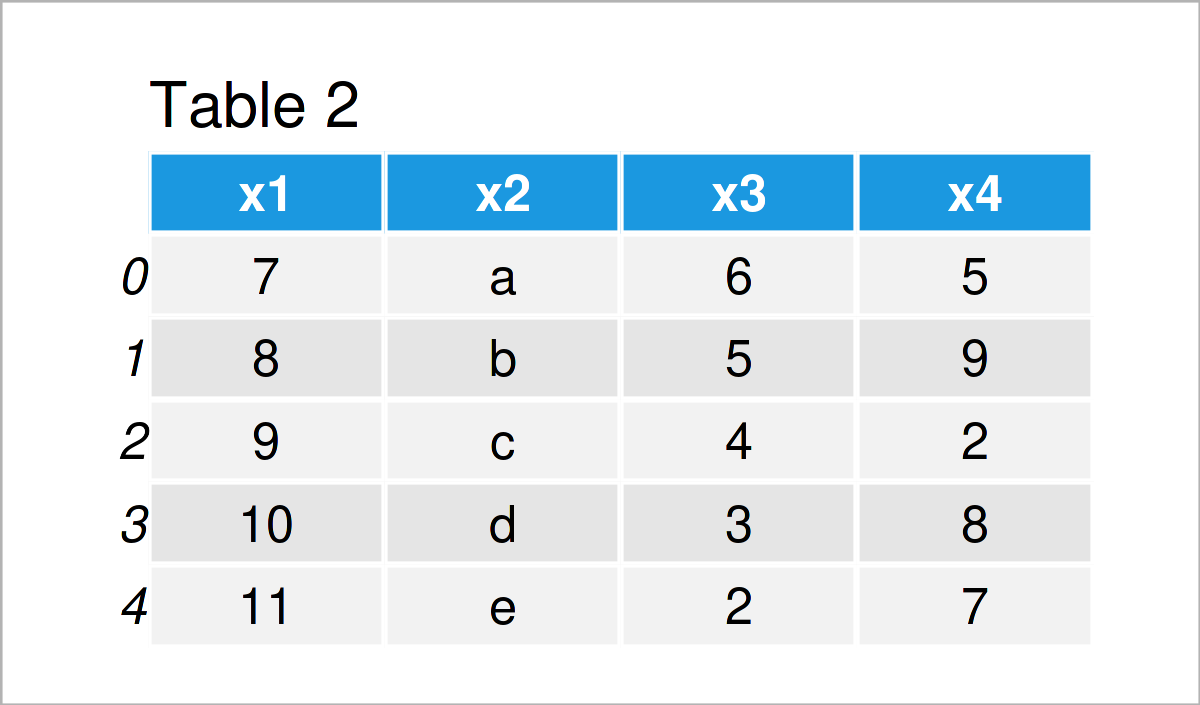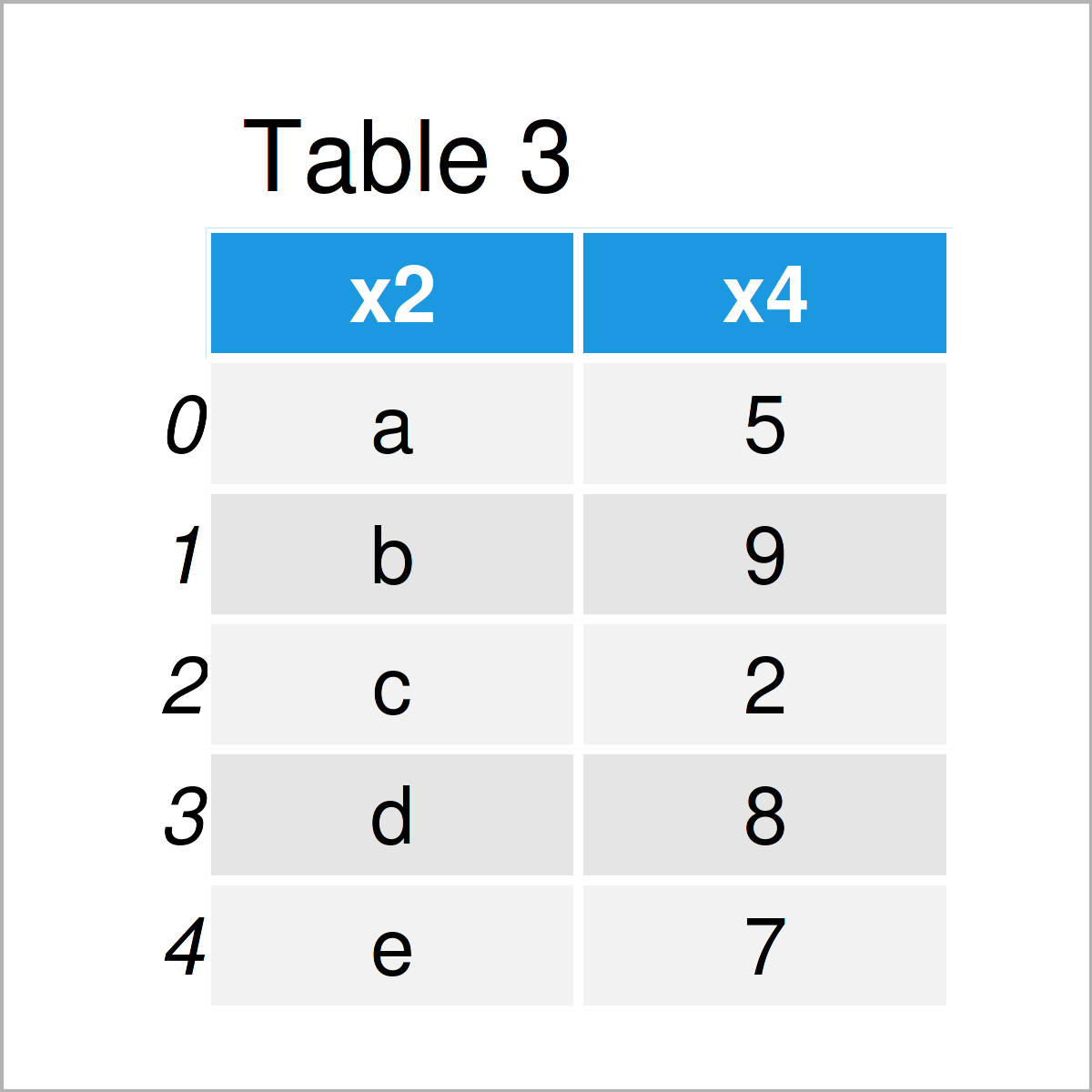# Create New pandas DataFrame from Existing Data in Python (2 Examples)

In this Python tutorial you’ll learn how to construct a new pandas DataFrame based on an existing data set.

The article looks as follows:

So without further additions, let’s jump right to the examples!

## Exemplifying Data & Libraries

In order to use the commands and functions of the pandas library, we first need to load pandas:

`import pandas as pd                        # Load pandas`

I’ll also have to create some example data:

```data = pd.DataFrame({'x1':range(7, 12),    # Create example DataFrame
'x2':['a', 'b', 'c', 'd', 'e'],
'x3':range(6, 1, - 1),
'x4':[5, 9, 2, 8, 7]})
print(data)                                # Print example DataFrame```As you can see based on Table 1, the example data is a DataFrame having five rows and four columns.

## Example 1: Create Copy of Entire pandas DataFrame

Example 1 illustrates how to create a duplicate of a pandas DataFrame.

For this task, we can use the copy() function as shown below:

```data_new1 = data.copy()                    # Create copy
print(data_new1)                           # Print copy```After running the previous Python programming code the new pandas DataFrame called data_new1 illustrated in Table 2 has been created.

As you can see, this DataFrame contains exactly the same variables and rows as our input data set.

## Example 2: Extract Specific Columns & Create New pandas DataFrame

In this example, I’ll demonstrate how to extract particular columns of a pandas DataFrame and save it in a new pandas DataFrame.

Once again, we can use the copy function. However, this time we also have to specify the columns that we want to select within double square brackets:

```data_new2 = data[['x2', 'x4']].copy()      # Extract selected columns
print(data_new2)                           # Print DataFrame subset```After running the previous Python programming syntax the pandas DataFrame subset shown in Table 3 has been created.

## Video & Further Resources

Do you need more info on the Python code of this tutorial? Then you might have a look at the following video on my YouTube channel. I demonstrate the Python code of this page in the video:

In addition, you might have a look at some of the other articles on statisticsglobe.com. You can find a selection of tutorials below.

On this page you have learned how to create a new pandas DataFrame based on an existing DataFrame in the Python programming language. In case you have further questions or comments, let me know in the comments.

Subscribe to the Statistics Globe Newsletter